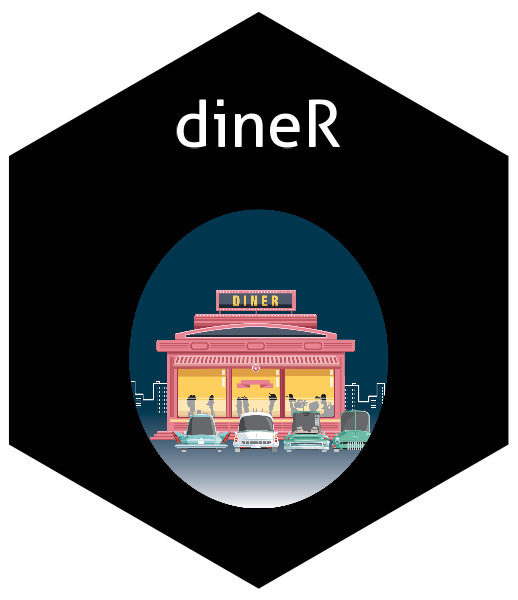# dineRThe goal of dineR is to enable users of all backgrounds to easily and computationally efficiently perform differential network estimation.

## Installation

You can install the released version of dineR from CRAN with:

``````install.packages("dineR")
``````

## Example

This is a basic example which shows you how to solve a common problem:

``````library(dineR)
# Data Generation
n_X <- 100
n_Y <- n_X
p_X <- 100
p_Y <- p_X
#case <- "sparse"
case <- "asymsparse"

data <- data_generator(n = n_X, p = p_X, seed = 123)

X <- data\$X
Y <- data\$Y
diff_Omega <- data\$diff_Omega
paste("The number of non-zero entries in the differential network is: ", sum(diff_Omega!=0))

# Estimation Preliminaries (All of the parameters are now optional as the function has pre-specified defaults)

loss <- "lasso"
nlambda <- 50
tuning <- "AIC"
stop_tol <- 1e-4
perturb <- F
correlation <- F
max_iter <- 500
lambda_min_ratio <- 0.5
#gamma <- 1 #Only if we use EBIC

# Estimation

result <- estimation(X, Y, loss = loss, nlambda = nlambda, tuning = tuning, stop_tol = stop_tol,
perturb = perturb, correlation = correlation,
max_iter = max_iter, lambda_min_ratio = lambda_min_ratio)

# Results

print(result\$path[][1:5,1:5])
result\$elapse
``````

## Try the dineR package in your browser

Any scripts or data that you put into this service are public.

dineR documentation built on Nov. 15, 2021, 5:09 p.m.